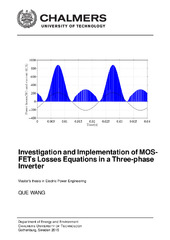## Investigation and Implementation of MOSFETs Losses Equations in a Three-phase Inverter

 dc.contributor.author Wang, Que dc.contributor.department Chalmers tekniska högskola / Institutionen för energi och miljö sv dc.contributor.department Chalmers University of Technology / Department of Energy and Environment en dc.date.accessioned 2019-07-03T13:52:18Z dc.date.available 2019-07-03T13:52:18Z dc.date.issued 2015 dc.description.abstract In this master thesis, the operation model of a three-phase MOSFET inverter and the corresponding current waveforms are analyzed. The mathematical expressions of the conduction losses based on the three-phase MOSFET inverter using PWM control method are presented. The algebraic equations are given with the parameters of a MOSFET-diode module, such as the on-state resistance, the body diode resistance and the voltage drop. Moreover, a numerical reference representation of the conduction losses is made to verify the analytical expressions. Numerical analysis is firstly based on the pure sinusoidal reference three-phase voltages. The results of the numerical analysis and the results of the analytical equations are coherent. A comparison of the MOSFET inverter with reverse conduction characteristics and the assumption without reverse conduction is made. Without reverse conduction, the losses of one power module are higher than the one with reverse conduction at both the high and low current levels. The difference can be more than 20 % compared to the case with reverse condition at the rated current of the selected module, CAS300M12BM2, 150 A. When the phase current is low and there is no diode conducting during the operation, the losses without reverse conduction can be more than 100 % higher. Furthermore, the losses resulting from third harmonic injection and using the common mode reduction are calculated, and compared with the losses using a pure sinusoidal reference. The conduction losses of those two different PWM methods are found to be small and presented in tables. dc.identifier.uri https://hdl.handle.net/20.500.12380/232013 dc.language.iso eng dc.setspec.uppsok LifeEarthScience dc.subject Elkraftteknik dc.subject Electric power engineering dc.title Investigation and Implementation of MOSFETs Losses Equations in a Three-phase Inverter dc.type.degree Examensarbete för masterexamen sv dc.type.degree Master Thesis en dc.type.uppsok H local.programme Electric power engineering (MPEPO), MSc